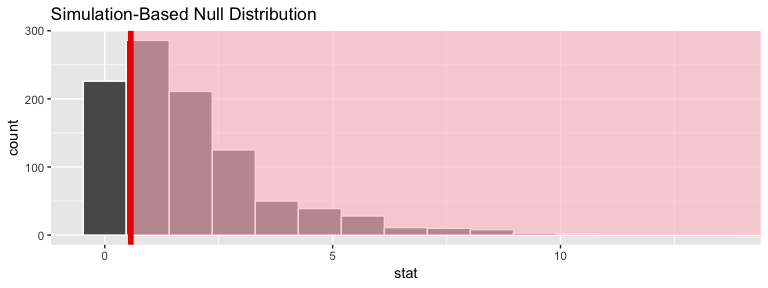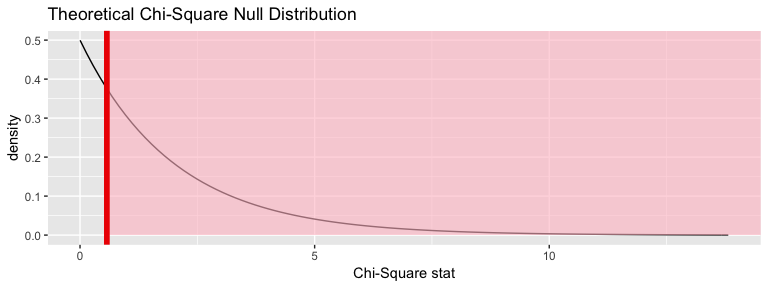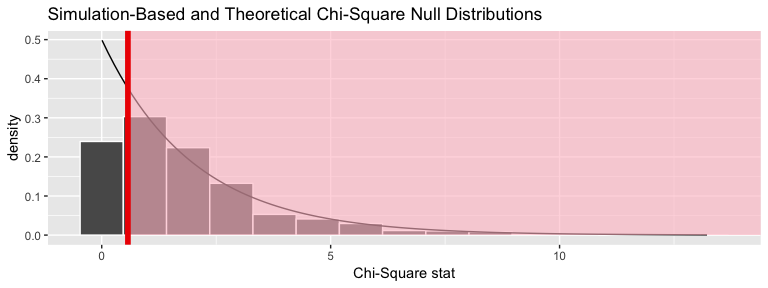# Chi-squared test example using nycflights13flights data

#### 2018-11-15

Note: The type argument in generate() is automatically filled based on the entries for specify() and hypothesize(). It can be removed throughout the examples that follow. It is left in to reiterate the type of generation process being performed.

## Data preparation

library(nycflights13)
library(dplyr)
library(ggplot2)
library(stringr)
library(infer)
set.seed(2017)
fli_small <- flights %>%
na.omit() %>%
sample_n(size = 500) %>%
mutate(season = case_when(
month %in% c(10:12, 1:3) ~ "winter",
month %in% c(4:9) ~ "summer"
)) %>%
mutate(day_hour = case_when(
between(hour, 1, 12) ~ "morning",
between(hour, 13, 24) ~ "not morning"
)) %>%
select(arr_delay, dep_delay, season,
day_hour, origin, carrier)
• Two numeric - arr_delay, dep_delay
• Two categories
• season ("winter", "summer"),
• day_hour ("morning", "not morning")
• Three categories - origin ("EWR", "JFK", "LGA")
• Sixteen categories - carrier

# One numerical variable, one categorical (2 levels)

## Calculate observed statistic

The recommended approach is to use specify() %>% calculate():

obs_chisq <- fli_small %>%
specify(origin ~ season) %>% # alt: response = origin, explanatory = season
calculate(stat = "Chisq")
The observed $$\chi^2$$ statistic is
stat
0.571898

.

Or using chisq_test in infer

obs_chisq <- fli_small %>%
chisq_test(formula = origin ~ season) %>%
dplyr::select(statistic)
Again, the observed $$\chi^2$$ statistic is
statistic
0.571898

.

Or using another shortcut function in infer:

obs_chisq <- fli_small %>%
chisq_stat(formula = origin ~ season)
Lastly, the observed $$\chi^2$$ statistic is
stat
0.571898

.

## Randomization approach to $$\chi^2$$-statistic

chisq_null_perm <- fli_small %>%
specify(origin ~ season) %>% # alt: response = origin, explanatory = season
hypothesize(null = "independence") %>%
generate(reps = 1000, type = "permute") %>%
calculate(stat = "Chisq")

visualize(chisq_null_perm) +
shade_p_value(obs_stat = obs_chisq, direction = "greater")## Calculate the randomization-based $$p$$-value

chisq_null_perm %>%
get_p_value(obs_stat = obs_chisq, direction = "greater")
p_value
0.748

## Theoretical distribution

chisq_null_theor <- fli_small %>%
specify(origin ~ season) %>%
hypothesize(null = "independence") %>%
# generate() ## Not used for theoretical
calculate(stat = "Chisq")

visualize(chisq_null_theor, method = "theoretical") +
shade_p_value(obs_stat = obs_chisq, direction = "right")
## Warning: Check to make sure the conditions have been met for the
## theoretical method. {infer} currently does not check these for you.## Overlay appropriate $$\chi^2$$ distribution on top of permuted statistics

visualize(chisq_null_perm, method = "both") +
shade_p_value(obs_stat = obs_chisq, direction = "right")
## Warning: Check to make sure the conditions have been met for the
## theoretical method. {infer} currently does not check these for you.## Compute theoretical p-value

fli_small %>%
chisq_test(formula = origin ~ season) %>%
dplyr::pull(p_value)
##  0.7513009